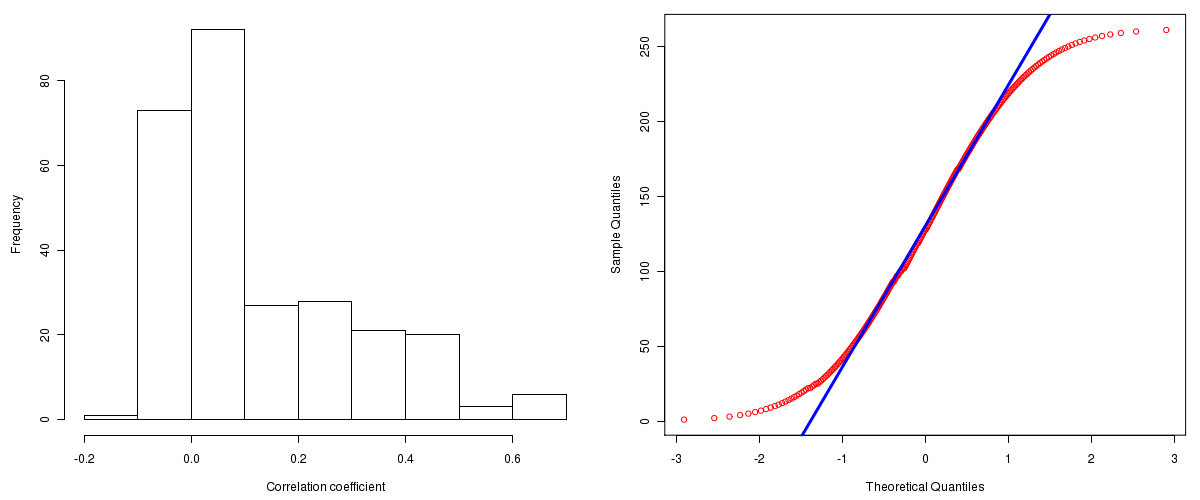Ovarian Serous Cystadenocarcinoma: Correlations between copy number and miR expression
Maintained by John Zhang (Dana-Farber Cancer Institute)
Overview
Introduction

A TCGA sample is profiled to detect the copy number variations and expressions of genes. This pipeline attempts to correlate copy number and expression data of genes across samples to determine if the copy number variations also result in differential expressions. This report contains the calculated correlation coefficients based on measurements of genomic copy number (log2) values and intensity of the expressions of the corresponding feature across patients. High positive/low negative correlation coefficients indicate that genomic alterations result in differences in the expressions of miRNA the genomic regions transcribe.

Summary

The correlation coefficients in 10, 20, 30, 40, 50, 60, 70, 80, 90 percentiles are -0.0365, -0.0124, 0.0053, 0.0249, 0.0542, 0.0968, 0.1851, 0.2803, 0.4009, respectively.

Results
Correlation results

Number of genes and samples used for the calculation are shown in Table 1. Figure 1 shows the distribution of calculated correlation coefficients and quantile-quantile plot of the calculated correlation coefficients against a normal distribution. Table 2 shows the top 20 features ordered by the value of correlation coefficients.

Table 1.  Counts of miR and number of samples in copy number and expression data sets and common to both

Category Copy number Expression Common
Sample 547 564 536
miR 299 822 299

Figure 1.  Summary figures. Left: histogram showing the distribution of the calculated correlations across samples for all miR. Right: QQ plot of the calculated correlations across samples. The QQ plot is used to plot the quantiles of the calculated correlation coefficients against that derived from a normal distribution. Points deviating from the blue line indicate deviation from normality.Table 2.  Get Full Table Top 20 features (defined by the feature column) ranked by correlation coefficients

feature r p-value q-value chrom start end geneid
hsa-miR-30e 0.6467 0 0 1 40992614 40992705 MI0000749
hsa-miR-30b 0.6351 0 0 8 135881945 135882032 MI0000441
hsa-miR-27a 0.6291 0 0 19 13808254 13808331 MI0000085
hsa-miR-185 0.6253 0 0 22 18400662 18400743 MI0000482
hsa-miR-30d 0.6069 0 0 8 135886301 135886370 MI0000255
hsa-miR-23a 0.6035 0 0 19 13808401 13808473 MI0000079
hsa-miR-641 0.5257 0 0 19 45480290 45480388 MI0003656
hsa-miR-17 0.5163 0 0 13 90800860 90800943 MI0000071
hsa-miR-484 0.508 0 0 16 15644652 15644730 MI0002468
hsa-miR-320 0.4822 0 0 8 22158420 22158501 MI0000542
hsa-miR-181d 0.4783 0 0 19 13846689 13846825 MI0003139
hsa-miR-200c 0.476 0 0 12 6943123 6943190 MI0000650
hsa-let-7b 0.4653 0 0 22 44888230 44888312 MI0000063
hsa-miR-186 0.4631 0 0 1 71305902 71305987 MI0000483
hsa-miR-106b 0.45 0 0 7 99529552 99529633 MI0000734
hsa-miR-148b 0.4387 0 0 12 53017267 53017365 MI0000811
hsa-miR-19a 0.4357 0 0 13 90801146 90801227 MI0000073
hsa-miR-25 0.4337 0 0 7 99529119 99529202 MI0000082
hsa-miR-27b 0.4283 0 0 9 96887548 96887644 MI0000440
hsa-miR-148a 0.4281 0 0 7 25956064 25956131 MI0000253
Methods & Data
Input

Gene level (TCGA Level III) expression data and copy number data of the corresponding loci derived by using the CNTools package of Bioconductor were used for the calculations. Pearson correlation coefficients were calculated for each pair of genes shared by the two data sets across all the samples that were common.

Correlation across sample

Pairwise correlations between the log2 copy numbers and expressions of each gene across samples were calculated using Pearson correlation.